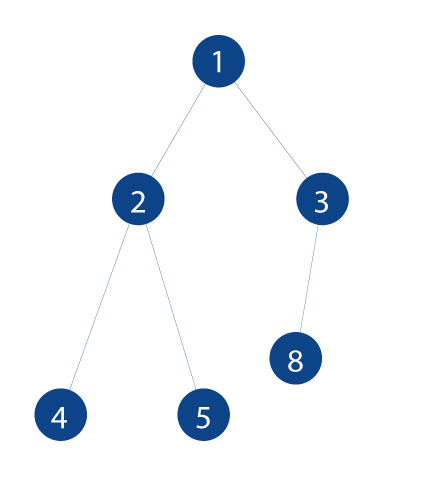## Posts

Showing posts with the label DS

### Tree data structure and its terminologyWhy we need a tree data structure? In the array, linked list, stack, and queue data is store in a sequential manner which is difficult to search specific data .the search becomes slow when a huge amount of data stored. This is a very annoying thing in the fast world. All people want to get the result as soon as possible. Different Tree data structures allow to access the data quickly and also it is not a linear data structure. First of all, look at the terminologies of the tree before understanding the concept of the tree. What is a tree? A collection of nodes that are connected by directed edges is known as tree. It is a nonlinear structure as compare to arrays, linked lists, and stacks. Node: A node is a struct that contains a value (data) and stores its pointer. There are two types of nodes internal nodes those nodes which contain at least one child leaf node and external nodes those nodes which are the last. Edge: This is a link between nodes. Root: It is the top most node of a tre

### circular queueCircular queue It is the type of queue in the data structure. The problem of the memory wastage faced in the normal queue is resolved in the circular.   How does a circular queue work? In this structure, we enqueue the value until the end of the queue is reached then again start from the front of the structure. The increment of the structure is to work on the module division with the size of the queue. Photo: Implementation of the circular queue:      Circular operations: Two pointer front and rear are used. Front is used to track the first element and initially, set value with -1. Rear is used to track the last element and initially, set value with -1. Enqueue operation: Firstly,check if the queue is full If not full then increase the value of the front from -1 to 0. Also, increase the value of the rear by 1, if the rear already pointed to the end then its next would be the start of the structure. Dequeue operation Firstly,check if the queue is empty or not. Return the value of the

### StackStack In this article, we will learn about the stack algorithm in the programming language.you will also learn about the operations and application of the algorithm Introduction of the stack: This is the abstract data type , look like the piles of the plates. The first plate remains in the last position in the piles. The last plate in the first position of the piles of the plates. If we want to take the first plate then we remove all the plates of the piles and find the first plate of the piles. The first in last out rule is followed in the piles of the plate. Same as in the stack algorithm. Operation of the stack:  The following are the operations(interface)provided by the algorithm. Push:  By push function, we can add the element on the top. Pop:  By pop function, we can get and remove the element from the top. IsEmpty: By IsEmpty we can check the stack is empty or not. IsFull: By IsFull we can check full or not. Implementation of the stack Data Member:

### Aysmptotic notationAsymptotic notation: In this article, we will learn about the Asymptotic notation: Big o notation, theta notation, and omega notation.  Asymptotic notations are the mathematical notations used to analyze the efficiency and effectiveness of the algorithm. The efficiency of the algorithm depends on the storage, amount of time, and many other factors. The algorithm does not have the same performance on different inputs. The performance change with the input. Why used asymptotic notations: The comparison of the algorithms is very important in the computer science world. There are a lot of algorithms but we want to choose the best one. The comparison of the algorithms is done by the asymptotic notations. let’s take an example of sorting, there are a lot of algorithms of the sorting selection sort, bubble sort, and merge sort,etc.we can use the best algorithm by the help of the asymptotic notations. Big o Notation: This tells about the worst case of the algorithm and the upper bound of the

### What is an algorithm